## Self Excitation:

Rather than arranging a separate dc source for excitation purposes, practical generators are always excited from their own armature terminals, this method of excitation being known as Self Excitation. A Self Excitation generator with connection as shown in Fig. 7.24 is known as a shunt generator by virtue of the parallel connection of the field winding with the armature.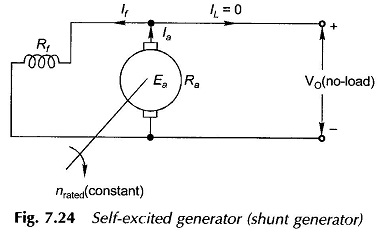Assume that the field is introduced into the circuit after the armature has been brought to rated speed. At the instant of switching on the field, the armature voltage corresponds to a small residual value which causes a small field current to flow. If the field is connected such that this current increases the field mmf and therefore the induced emf, the machine will continuously build-up. This indeed is a positive feedback connection and the machine builds up to a fine steady value only because of the saturation characteristic of the machine’s magnetic circuit.

Since the generator is assumed to be on no-load during the build-up process, the following circuit relationships apply (Fig. 7.24).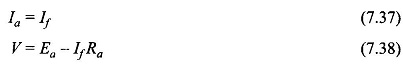The field current in a shunt generator being very small, the voltage drop /fRa can be neglected so that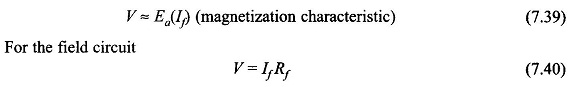which is a straight line relationship, called the Rf-line, in V-/f plot of Fig. 7.25.

The no-load terminal voltage is the solution of Eqs (7.39) and (7.40). Thus the intersection point P of the R1line with the magnetization characteristic as shown in Fig. 7.25 gives the no-load terminal voltage (V0) and the corresponding field current. Further, it is easy to visualize from Fig. 7.25 that the no-load voltage can be adjusted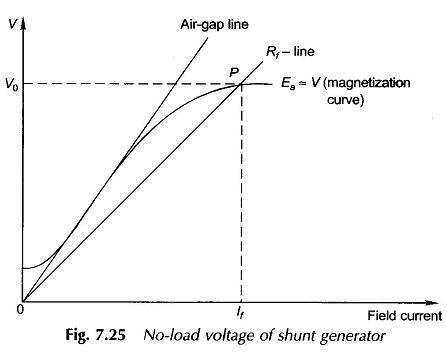to a desired value by changing the field resistance. It can also be seen with reference to Fig. 7.26 that as the field resistance is increased the no-load voltage decreases. The no-load voltage is undefined for a field resistance (Rf,, =Rfe) whose line coincides with the linear portion of the magnetization curve. With field resistance even slightly more than this value, the machine does not excite to any appreciable value and would give no-load voltage close to the residual value. The machine with this much resistance in the field fails to excite and the corresponding resistance is known as the critical resistance (Rf).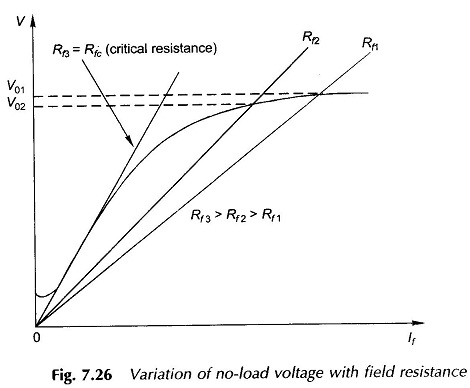Consider now the operation with fixed Rf and variable armature speed. It is observed from Fig. 7.27 that as the speed is reduced the OCC proportionally slides downwards so that the no-load voltage reduces. At a particular speed, called the critical speed, the OCC is tangential to the Rf -line and as a result the generator would fail to excite.

To summarize, a dc shunt generator may fail to self-excite for any of the three reasons mentioned below: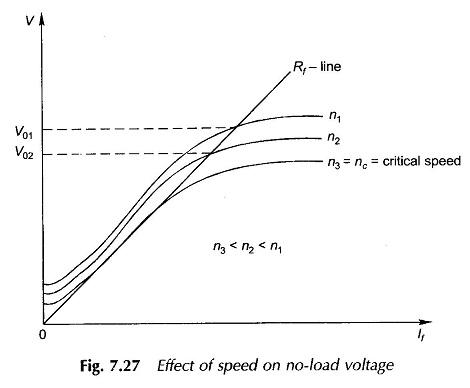1. Residual magnetism is absent.
2. The field connection to the armature is such that the induced emf due to the residual magnetism tends to destroy the residual magnetism (i.e. the feedback is negative).
3. The field circuit resistance is more than the critical value.

Condition (2) can be remedied simply by reversing the field connection to the armature or reversing the direction of rotation. In large dc generators with permanent connections and a fixed direction of rotation, the problem is overcome by temporarily exciting the field from a battery source. (This is known as flashing.)